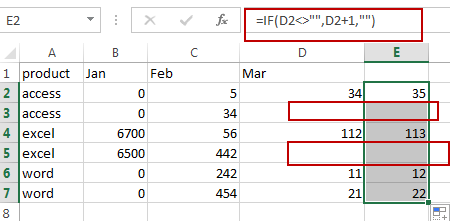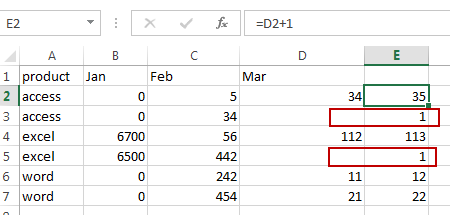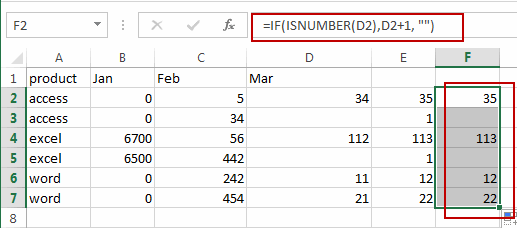# How to Ignore Blank Cells in a Formula in Excel

This post will guide you how to ignore blank cells in a formula in Excel. How do I ignore blank cells when performing calculations in a formula in Excel.

## Ignore Blank Cells in a Formula

If you have a formula to calculate a range of cells, and you want to ignore the blank cells. And you can use the IF function, the ISNUMBER function, the AND or OR function to achieve the result.

For example, you want to add 1 to all cells in range D2:D7, and if you directly use the formula =D2+1, and the blank cells also will be added 1. So if you want to ignore all blank cells in this formula, you need to use IF function to check if it is a blank cell, if TRUE, then ignore it. Otherwise, apply this formula. Just use the following formula:

`=IF(D2<>"",D2+1, "")`You can also use the IF function in combination with the ISNUMBER function to achieve the same result. Like this:

`=IF(ISNUMBER(D2),D2+1, "")`Video：Ignore Blank Cells in a Formula

### Related Functions

• Excel ISNUMBER function
The Excel ISNUMBER function returns TRUE if the value in a cell is a numeric value, otherwise it will return FALSE.The syntax of the ISNUMBER function is as below:= ISNUMBER (value)…
• Excel IF function
The Excel IF function perform a logical test to return one value if the condition is TRUE and return another value if the condition is FALSE.The syntax of the IF function is as below:= IF (condition, [true_value], [false_value])…

Sidebar